# Frank solutions for Class 9 Maths ICSE chapter 17 - Pythagoras Theorem [Latest edition]

#### Chapters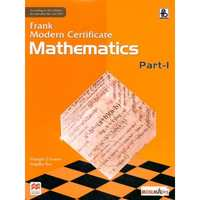## Chapter 17: Pythagoras Theorem

Exercise 17.1
Exercise 17.1

### Frank solutions for Class 9 Maths ICSE Chapter 17 Pythagoras Theorem Exercise 17.1

Exercise 17.1 | Q 1

Find the length of the perpendicular of a triangle whose base is 5cm and the hypotenuse is 13cm. Also, find its area.

Exercise 17.1 | Q 2

Find the length of the hypotenuse of a triangle whose other two sides are 24cm and 7cm.

Exercise 17.1 | Q 3

Calculate the area of a right-angled triangle whose hypotenuse is 65cm and one side is 16cm.

Exercise 17.1 | Q 4

A man goes 10 m due east and then 24 m due north. Find the distance from the straight point.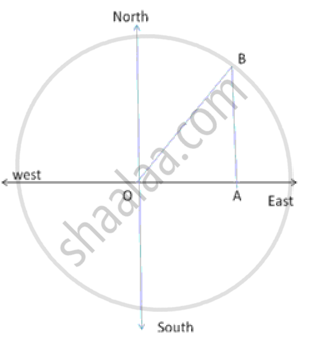Exercise 17.1 | Q 5

A ladder 25m long reaches a window of a building 20m above the ground. Determine the distance of the foot of the ladder from the building.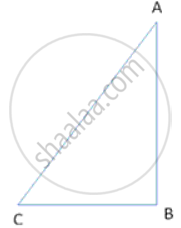Exercise 17.1 | Q 6

A right triangle has hypotenuse p cm and one side q cm. If p - q = 1, find the length of third side of the triangle.

Exercise 17.1 | Q 7

A ladder 15m long reaches a window which is 9m above the ground on one side of a street. Keeping its foot at the same point, the ladder is turned to other side of the street to reach a window 12m high. Find the width of the street.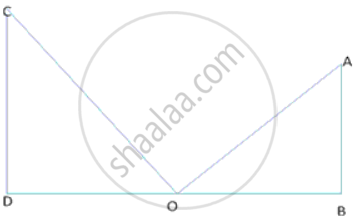Exercise 17.1 | Q 8

The foot of a ladder is 6m away from a wall and its top reaches a window 8m above the ground. If the ladder is shifted in such a way that its foot is 8m away from the wall to what height does its tip reach?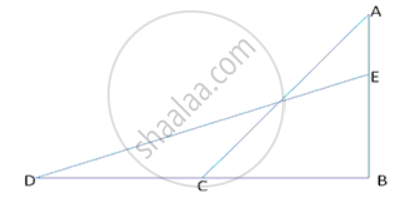Exercise 17.1 | Q 9

Two poles of height 9m and 14m stand on a plane ground. If the distance between their 12m, find the distance between their tops.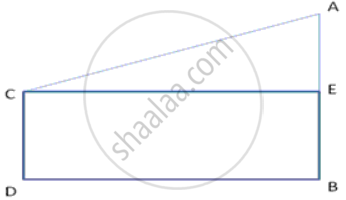Exercise 17.1 | Q 10

The length of the diagonals of rhombus are 24cm and 10cm. Find each side of the rhombus.

Exercise 17.1 | Q 11

Each side of rhombus is 10cm. If one of its diagonals is 16cm, find the length of the other diagonals.

Exercise 17.1 | Q 12

In ΔABC, AD is perpendicular to BC. Prove that: AB2 + CD2 = AC2 + BD2

Exercise 17.1 | Q 13

In an equilateral triangle ABC, the side BC is trisected at D. Prove that 9 AD2 = 7 AB2.

Exercise 17.1 | Q 14.1

From a point O in the interior of aΔABC, perpendicular OD, OE and OF are drawn to the sides BC, CA and AB respectively. Prove that: AF2 + BD2 + CE= OA2 + OB2 + OC2 - OD2 - OE2 - OF2

Exercise 17.1 | Q 14.2

From a point O in the interior of aΔABC, perpendicular OD, OE and OF are drawn to the sides BC, CA and AB respectively. Prove that: AF2 + BD2 + CE2 = AE2 + CD2 + BF2

Exercise 17.1 | Q 15.1

In a triangle ABC, AC > AB, D is the midpoint BC, and AE ⊥ BC. Prove that: AC2 = AD2 + BC x DE + (1)/(4)"BC"^2

Exercise 17.1 | Q 15.2

In a triangle ABC, AC > AB, D is the midpoint BC, and AE ⊥ BC. Prove that: AB2 = AD2 - BC x CE + (1)/(4)"BC"^2

Exercise 17.1 | Q 15.3

In a triangle ABC, AC > AB, D is the midpoint BC, and AE ⊥ BC. Prove that: AB2 + AC2 = 2AD2 + (1)/(2)"BC"^2

Exercise 17.1 | Q 15.4

In a triangle ABC, AC > AB, D is the midpoint BC, and AE ⊥ BC. Prove that: AC2 - AB2 = 2BC x ED

Exercise 17.1 | Q 15.5

In a triangle ABC, AC > AB, D is the midpoint BC, and AE ⊥ BC. Prove that: AB2 + AC2 = 2(AD2 + CD2)

Exercise 17.1 | Q 16

A point OI in the interior of a rectangle ABCD is joined with each of the vertices A, B, C and D. Prove that  OB2 + OD2 = OC2 + OA2

Exercise 17.1 | Q 17

ABCD is a rhombus. Prove that AB2 + BC2 + CD2 + DA2= AC2 + BD2

Exercise 17.1 | Q 18

AD is perpendicular to the side BC of an equilateral ΔABC. Prove that 4AD2 = 3AB2.

Exercise 17.1 | Q 19

The perpendicular AD on the base BC of a ∆ABC intersects BC at D so that DB = 3 CD. Prove that 2"AB"^2 = 2"AC"^2 + "BC"^2

Exercise 17.1 | Q 20.1

In a triangle ABC right angled at C, P and Q are points of sides CA and CB respectively, which divide these sides the ratio 2 : 1.
Prove that: 9AQ2 = 9AC2 + 4BC2

Exercise 17.1 | Q 20.2

In a triangle ABC right angled at C, P and Q are points of sides CA and CB respectively, which divide these sides the ratio 2 : 1.
Prove that: 9BP2 = 9BC2 + 4AC2

Exercise 17.1 | Q 20.3

In a triangle ABC right angled at C, P and Q are points of sides CA and CB respectively, which divide these sides the ratio 2 : 1.
Prove that : 9(AQ2 + BP2) = 13AB2

Exercise 17.1 | Q 21

In the given figure, PQ = "RS"/(3) = 8cm, 3ST = 4QT = 48cm.
SHow that ∠RTP = 90°.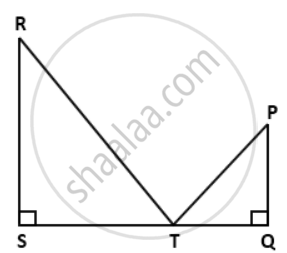Exercise 17.1 | Q 22

In a right-angled triangle ABC,ABC = 90°, AC = 10 cm, BC = 6 cm and BC produced to D such CD = 9 cm. Find the length of AD.

Exercise 17.1 | Q 23

In the given figure. PQ = PS, P =R = 90°. RS = 20 cm and QR = 21 cm. Find the length of PQ correct to two decimal places.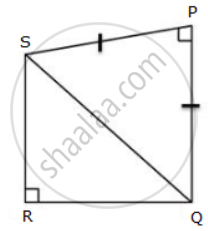Exercise 17.1 | Q 24

In a right-angled triangle PQR, right-angled at Q, S and T are points on PQ and QR respectively such as PT = SR = 13 cm, QT = 5 cm and PS = TR. Find the length of PQ and PS.

Exercise 17.1 | Q 25

PQR is an isosceles triangle with PQ = PR = 10 cm and QR = 12 cm. Find the length of the perpendicular from P to QR.

Exercise 17.1 | Q 26

In a square PQRS of side 5 cm, A, B, C and D are points on sides PQ, QR, RS and SP respectively such as PA = PD = RB = RC = 2 cm. Prove that ABCD is a rectangle. Also, find the area and perimeter of the rectangle.

## Chapter 17: Pythagoras Theorem

Exercise 17.1## Frank solutions for Class 9 Maths ICSE chapter 17 - Pythagoras Theorem

Frank solutions for Class 9 Maths ICSE chapter 17 (Pythagoras Theorem) include all questions with solution and detail explanation. This will clear students doubts about any question and improve application skills while preparing for board exams. The detailed, step-by-step solutions will help you understand the concepts better and clear your confusions, if any. Shaalaa.com has the CISCE Class 9 Maths ICSE solutions in a manner that help students grasp basic concepts better and faster.

Further, we at Shaalaa.com provide such solutions so that students can prepare for written exams. Frank textbook solutions can be a core help for self-study and acts as a perfect self-help guidance for students.

Concepts covered in Class 9 Maths ICSE chapter 17 Pythagoras Theorem are Regular Polygon, Right-angled Triangles and Pythagoras Property, Right-angled Triangles and Pythagoras Property.

Using Frank Class 9 solutions Pythagoras Theorem exercise by students are an easy way to prepare for the exams, as they involve solutions arranged chapter-wise also page wise. The questions involved in Frank Solutions are important questions that can be asked in the final exam. Maximum students of CISCE Class 9 prefer Frank Textbook Solutions to score more in exam.

Get the free view of chapter 17 Pythagoras Theorem Class 9 extra questions for Class 9 Maths ICSE and can use Shaalaa.com to keep it handy for your exam preparation